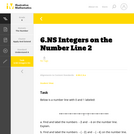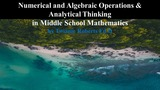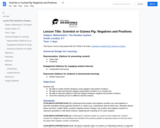Updating search results...

# 13 Results

View
Selected filters:
• MCCRS.Math.Content.6.NS.C.6aUnrestricted Use
CC BY
Rating
0.0 stars

This is a task from the Illustrative Mathematics website that is one part of a complete illustration of the standard to which it is aligned. Each task has at least one solution and some commentary that addresses important aspects of the task and its potential use.

Subject:
Mathematics
Numbers and Operations
Material Type:
Activity/Lab
Provider:
Illustrative Mathematics
Provider Set:
Illustrative Mathematics
Author:
Illustrative Mathematics
08/06/2015Conditional Remix & Share Permitted
CC BY-SA
Rating
0.0 stars

This resource links to both the Fractions progression document published by the Common Core Writing Teams in June 2011 and the Module posted on the Illustrative Mathematics website.

Material Type:
Assessment
Homework/Assignment
Teaching/Learning Strategy
08/08/2019Educational Use
Rating
0.0 stars

In this video from Cyberchase, Harry must score a total of 50 points in various events to qualify for the Northern Hemisphere games in New Orleans.

Subject:
Mathematics
Material Type:
Lecture
Provider:
PBS LearningMedia
Provider Set:
PBS Learning Media: Multimedia Resources for the Classroom and Professional Development
Author:
U.S. Department of Education
WNET
07/24/2008Unrestricted Use
CC BY
Rating
0.0 stars

This lesson will be the first of six lessons guiding students in constructing the abstract concepts necessary to understand adding positive and negative integers.
It is designed for adult learners (or middle school or older) who are not fluent with using numbers.

Subject:
Mathematics
Material Type:
Lesson Plan
06/08/2018Educational Use
Rating
0.0 stars

In this Cyberchase video segment, the CyberSquad locates the Cyberchase Council by using negative numbers.

Subject:
Mathematics
Material Type:
Lecture
Provider:
PBS LearningMedia
Provider Set:
PBS Learning Media: Multimedia Resources for the Classroom and Professional Development
Author:
U.S. Department of Education
WNET
07/24/2008Conditional Remix & Share Permitted
CC BY-NC
Rating
0.0 stars
Rating
0.0 stars
Subject:
Mathematics
Material Type:
Full Course
Provider:
Pearson
10/06/2016Conditional Remix & Share Permitted
CC BY-NC
Rating
0.0 stars

Rational Numbers

Type of Unit: Concept

Prior Knowledge

Students should be able to:

Solve problems with positive rational numbers.
Plot positive rational numbers on a number line.
Understand the equal sign.
Use the greater than and less than symbols with positive numbers (not variables) and understand their relative positions on a number line.
Recognize the first quadrant of the coordinate plane.

Lesson Flow

The first part of this unit builds on the prerequisite skills needed to develop the concept of negative numbers, the opposites of numbers, and absolute value. The unit starts with a real-world application that uses negative numbers so that students understand the need for them. The unit then introduces the idea of the opposite of a number and its absolute value and compares the difference in the definitions. The number line and positions of numbers on the number line is at the heart of the unit, including comparing positions with less than or greater than symbols.

The second part of the unit deals with the coordinate plane and extends student knowledge to all four quadrants. Students graph geometric figures on the coordinate plane and do initial calculations of distances that are a straight line. Students conclude the unit by investigating the reflections of figures across the x- and y-axes on the coordinate plane.

Subject:
Mathematics
Numbers and Operations
Material Type:
Unit of Study
Provider:
PearsonConditional Remix & Share Permitted
CC BY-NC
Rating
0.0 stars

Students revise their work on the Self Check based on feedback from the teacher and their peers.Key ConceptsConcepts from previous lessons are integrated into this assessment task: integers, absolute value, and comparing numbers. Students apply their knowledge, review their work, and make revisions based on feedback from the teacher and their peers. This process creates a deeper understanding of the concepts.Goals and Learning ObjectivesApply your knowledge of integers, absolute value, and comparing numbers to solve problems.Track and review your choice of strategy when problem solving.

Subject:
Numbers and Operations
Material Type:
Lesson Plan
09/21/2015Conditional Remix & Share Permitted
CC BY-NC
Rating
0.0 stars

Students watch a dot get tossed from one number on a number line to the opposite of the number. Students predict where the dot will land each time based on its starting location.Key ConceptsThe opposite of a number is the same distance from 0 as the number itself, but on the other side of 0 on a number line.In the diagram, m is the opposite of n, and n is the opposite of m. The distance from m to 0 is d, and the distance from n to 0 is d; this distance to 0 is the same for both n and m. The absolute value of a number is its distance from 0 on a number line.Positive numbers are numbers that are greater than 0.Negative numbers are numbers that are less than 0.The opposite of a positive number is negative, and the opposite of a negative number is positive.Since the opposite of 0 is 0 (which is neither positive nor negative), then&nbsp;0 = 0. The number 0 is the only number that is its own opposite.Whole numbers and the opposites of those numbers are all integers.Rational numbers are numbers that can be expressed as ab, where a and b are integers and b &ne; 0.Goals and Learning ObjectivesIdentify a number and its oppositeLocate the opposite of a number on a number lineDefine the opposite of a number, negative numbers, rational numbers, and integers

Subject:
Numbers and Operations
Material Type:
Lesson Plan
09/21/2015Conditional Remix & Share Permitted
CC BY-NC
Rating
0.0 stars

Students analyze whether given statements are possible or impossible using their definitions of absolute value and the opposite of a number. If the statements are possible, students give an example of a pair of numbers that fit the statement. If the statements are impossible, students explain why.Key ConceptsA number and the opposite of the number always have the same absolute value.In general, taking the opposite of n changes the sign of n. For example, the opposite of 3 is &minus;3.In general, taking the absolute value of n gives a number |n|, which is always positive. For example, |3| = 3 and |&minus;3| = 3.Since the opposite of 0 is 0 (which is neither positive nor negative), therefore &minus;0 = 0. The number 0 is the only number which is its own opposite.Goals and Learning ObjectivesFind pairs of numbers that satisfy different statements about absolute values and/or the opposites of numbers.State when it is impossible to find a pair of numbers that satisfies the statement and explain why.

Subject:
Numbers and Operations
Material Type:
Lesson Plan
09/21/2015Unrestricted Use
CC BY
Rating
0.0 stars

This supplemental resource provides problems and activities related to&nbsp;Numerical and Algebraic Operations &amp; Analytical Thinking in Middle School Mathematics.

Subject:
Mathematics
Material Type:
Activity/Lab
Author:
Twianie Roberts Ed.D
09/25/2022Some Rights Reserved
Rating
0.0 stars

Navigate the number line while diving amidst shipwrecks and sunken ruins. Will you find a pearl, or an old boot? Watch out for the electric eel! Pearl Diver teaches properties of numbers, how to plot numbers, how to visualize quantity on the number line, how to order numbers, and how to use the number line as a visual model for mathematical operations.

Subject:
Mathematics
Material Type:
Game
Interactive
Provider:
Learning Games Lab
Author:
NMSU Learning Games Lab
07/20/2015Conditional Remix & Share Permitted
CC BY-NC
Rating
0.0 stars

Students explore the concepts of positive and negative numbers through Newton's Third Law and rocket thrust, and other examples.

Subject:
Mathematics
Material Type:
Lesson Plan
Provider:
National Air and Space Museum
Author:
National Air and Space Museum